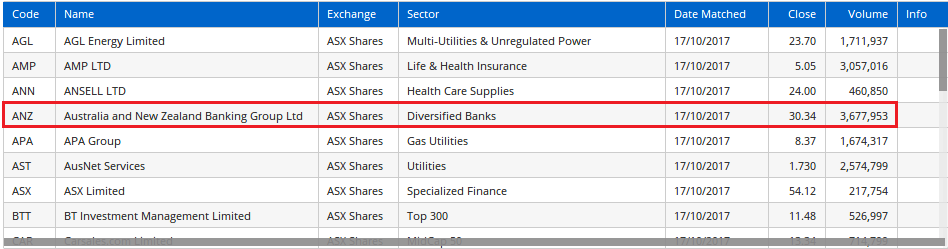# ISTICKER() Function

Author: Optuma Team Last updated: Feb 7, 2019 01:59

## Overview

The `ISTICKER()` function allows a specific criteria to be used on a set source code only. If the source code does not match the script criteria will not be applied. This is useful when you want to use a script in an area like scanning, but have some criteria items specific to one code.

For example:

``````GS1 = GannSwing() ISDOWN;
MA1 = MA(BARS=20);
MA2 = MA(BARS=120);
((MA1 > MA2) and ISTICKER(CODE=ANZ:ASX)) OR GS1
``````

If a script containing the above was used in a scan across the ASX, the criteria regarding the Moving Averages would only apply to ANZ.All other codes in the scan would disregard this specific criteria and only use the Gann Swing criteria to pass / fail the scan.

We can also specify another set of criteria for other specific codes by using the OR operator. The following criteria restricts to only look for ANZ and CBA. CBAs criteria refers to ATR, ANZ refers to Moving Averages.

``````MA1 = MA(BARS=20);
MA2 = MA(BARS=120);
((MA1 > MA2) and ISTICKER(CODE=ANZ:ASX)) OR
((ISTICKER(CODE=CBA:ASX)) and (ATR() &gt; 1))
``````

### Setting up a Binary Trade Back Test

As seen in this blog article, it’s possible to test a binary trading system, ie buy stocks when relatively stronger than bonds, and the reverse when bonds are stronger than stocks. This is done using the `ISTICKER()` function:

``````// Calculate the SPY/TLT ratio
S1=GETDATA(CODE=SPY:US);
T1=GETDATA(CODE=TLT:US);
Ratio=S1/T1;

//Calculate the moving averages of the ratio
MA10=MA(Ratio,BARS=10,CALC=Close);
MA50=MA(Ratio,BARS=50,CALC=Close);

//When 10MA crosses above 50MA buy SPY, or buy TLT if crosses below
MA10 CrossesAbove MA50 and ISTICKER(CODE=SPY:US) or
MA10 CrossesBelow MA50 and ISTICKER(CODE=TLT:US)\*\*
``````